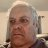# Creating ATR and Gap labels

S

#### sawyersweetman

##### New member
Hello,

I am trying to make Labels for my charts. ATR and GAP labels.

Problem is my labels are using the Intraday data, I would like to see the information for the daily data. Daily ATR and Daily Gap.

I just need it to pull the daily aggregated information not sure how to do that.
i just want to see the daily gap and daily ATR on the intraday chart and not have the chart data effect the output.

If someone can please help me code this that would be very much appreciated. the frustration is real. Current code below

Current Gap label

plot Gap = round((open-close) / open*100);

Gap.assignValueColor (if Gap>0 then color.LIGHT_GREEN else color.LIGHT_RED);

AddLabel(yes, Gap + "%", color = Color.WHITE);

Current ATR label
input ATRLength = 14;

def ATR = Round(Average(TrueRange(high, close, low), ATRLength), 2);
def iv = Round(close() * (imp_volatility() / 15.87), 3);

Last edited by a moderator:
G

#### generic

##### New member
@sawyersweetman

Code:
``````input agg = AggregationPeriod.DAY;
def open_agg = open(period = agg);
def high_agg = high(period = agg);
def low_agg = low(period = agg);
def close_agg= close(period = agg);
def iv_agg = imp_volatility(period = agg);

#Current Gap label
plot Gap = round((open_agg-close_agg) / open_agg*100);

Gap.assignValueColor (if Gap>0 then color.LIGHT_GREEN else color.LIGHT_RED);

AddLabel(yes, Gap + "%", color = Color.WHITE);

#Current ATR label
input ATRLength = 14;

def ATR = Round(Average(TrueRange(high_agg, close_agg, low_agg), ATRLength), 2);
def iv = Round(close_agg * (iv_agg / 15.87), 3);

S

#### sawyersweetman

##### New member
@generic SIICK! Thanks so much!!Creating a counter with variable input Questions 4